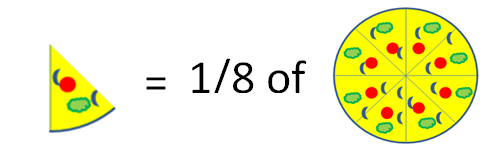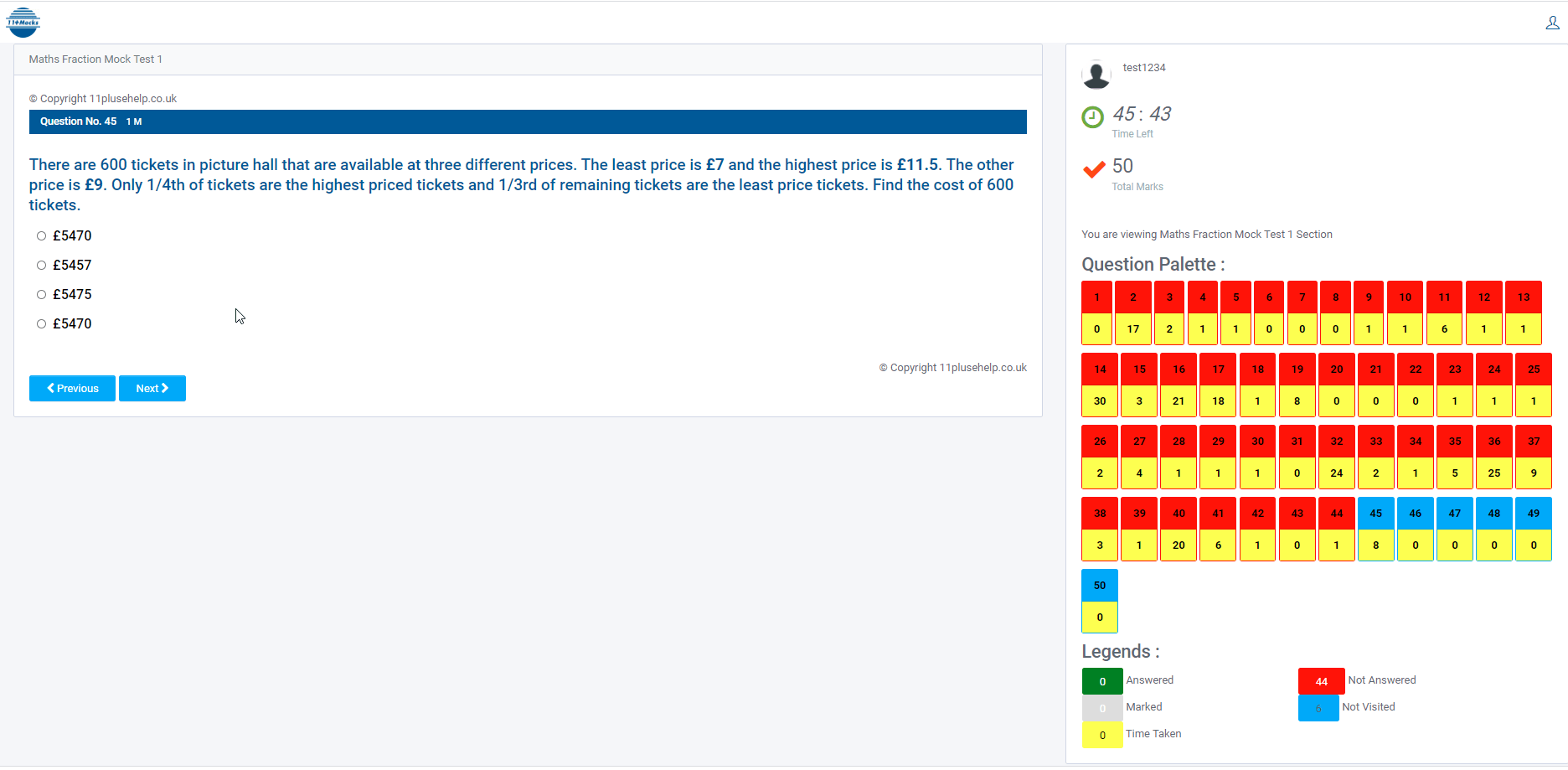# 11 Plus Fractions Mock Test

## 11 Plus Fractions Mock Test

Please register at11plus-mockexams.co.uk to take the 11 Plus Fractions Mock Test :

https://11plus-mockexams.co.uk/

After login, go to the “Topic-wise Mocks” menu and click the “Start” button against test “Maths Fractions Mock Test 1” to take the 11 Plus Fractions Mock Test.

How to take 11 Plus Mock Exams:

How to take 11 Plus Mock Tests Online

11plus-mockexams.co.uk has developed a unique exclusive feedback reporting system to provide subject wise Mock Results and a percentile ranking of your child compared to the cohort that sat the test. Mock Result is the mirror of your 11plus preparation. So we pay much more attention to analyse your results.

## 11 Plus Fractions Mock Test:

All these tests lead to perfect practice, and the child should be fully prepared to face any 11 Plus Grammar School tests or Independent Schools tests.

These tests are useful for preparing CEM, CSSE, GL Assessment, Grammar Schools, Independent Schools and any other 11 Plus entrance examinations ( Grammar schools or Independent schools) in the UK.

#### Fractions:

Fractions are considered as representing a part of a whole quantity. Fractions are usually represented in the form of a/b where a, b are 0. The terminologies of fractions are generally used while dividing a thing into smaller parts, like sharing a pizza among friends etc.The pizza shown in the above picture has been divided into eight pieces. When the pizza is considered a whole, then each part is given by 1/8, which is a fraction.A fraction consists of 2 parts they are Numerator and Denominator.

## FREE 11 Plus Percentages Mock Test:

FREE 11 Plus Percentages Mock Test

### 11 Plus Fractions Syllabus:

Count up and down in hundredths; recognise that hundredths arise when dividing an object
by a hundred and dividing tenths by ten.
Solve problems involving increasingly harder fractions to calculate quantities and fractions
to divide quantities, including non-unit fractions where an answer is a whole number
identify, name and write equivalent fractions of a given fraction, including tenths and
hundredths
Add and subtract fractions with the same denominator

Compare and order fractions whose denominators are all multiples of the same number
Recognise mixed numbers and improper fractions and convert from one form to the other
Add and subtract fractions with the same denominator and related fractions; write
mathematical statements >1 as a mixed number (e.g. 2/5 + 4/5 = 6/5 = 11/5) 26
Multiply proper fractions and mixed numbers by whole numbers, supported by materials
and diagrams.

Use common factors to simplify fractions; use common multiples to express fractions in the
same denomination
Compare and order fractions, including fractions >1
Associate a fraction with the division to calculate decimal fraction equivalents (e.g. 0.375) for a
simple fraction (e.g. 3/8).
Add and subtract fractions with different denominators and mixed numbers, using the
concept of equivalent fractions
Multiply simple pairs of proper fractions, writing the answer in its simplest form (e.g. 1/4 ×
1/2 = 1/8)
Divide proper fractions by whole numbers (e.g. 1/3 ÷ 2 = 1/6 ).

We provided different questions in all possible models and covered the 11 Plus Fractions topic.

11 Plus CEM Mock Paper 1

Sample Report:Check out our article on 11 Plus Mock Exams Website Features to know more about it:

11 Plus-MockExams website Features

You can see the 11 Plus Mock Exams Results Report here:

11 Plus Mock Exams Results ReportGenerally, fractions can be divided into three types they are

• Proper Fractions
• Improper fractions
• Mixed Fractions

The fractions in which the denominator is greater than the numerator are considered the Proper Fractions. Examples are ½, ¼, ¾, etc.

The fractions in which the denominator is greater than the numerator are considered Improper Fractions. Examples are 11/4, 5/2, 10/7 etc

The other form of representing an improper fraction is in terms of Mixed Fractions. It is a combination of a whole number and a proper fraction. Examples are 3 ½, 5 ¼, 7 ¾, etc.

Some important facts about fractions are

• The fractions with the same denominator are Like Fractions else they are said as, Unlike Fractions.
• A fraction in which numerator and denominator are equal then its value will be equal to One. Eg 2/2 = 1.
• A fraction in which the numerator = 0 then the value of the fraction will be Zero. Eg 0/100 = 0.
• A fraction in which the denominator = 0 then the fraction is said to be undefined.

Example: Jason brings a cake and cuts it into 6 equal parts and distributes among his friend, what fraction does each friend get?

Sol: No. of pieces = 6 then each one will be getting 1/6 part of the cake.

11 Plus Mock Exam Papers

11 Plus Mock Exam Papers

Arithmetic operations on fractions

To perform addition or subtraction, we need to check if the fractions have the same denominator or not. We will know about them in detail through some interesting and easy examples.

Consider two fractions a/b and c/b then their sum will be (a + c)/b, similarly in subtraction also, it will be (a-c)/b. Here denominators of both the fractions are equal.

Consider two fractions a/b and c/d then to find their sum or difference we must make their denominators equal. For this we must find the LCM of the denominators and based on it we must make the fractions like or we follow another method, in which the fractions are represented as (ad/bd), (bc/bd) and perform the addition or subtraction.

Sol: First convert them into like fractions. 1/2 can be written as (1×3) / (2×3) = 3/6. Similarly, 2/3 can be written as 2/6. Now we can add both the fractions,

3/6 + 2/6 = 5/6

For multiplication it is very easy, just multiply the numerators and denominators separately. For division just reciprocate the 2nd fraction and multiply.

Multiplication of a / b & c / d = (a x b) / (c x d)

Division of a / b & c / d = (a x d) / (b x c)

Example: Multiply 1/4 and 2/3.

Sol: It is very easy just to multiply numerators and denominators separately.

1/4 x 2/3 = (1 x 2) / (4 x 3) = 2 / 12.

11plus-mockexams.co.uk system is powered to identify areas of weaknesses of the children who sat the exam. This system will help you to understand the capabilities and weaknesses of your child. This way, you can understand the areas or subjects your children need to improve.

Some of the examples from 11plus-mockexams.co.uk:We focus on fundamentals, logic, basics and cover most of the syllabus for all 11 Plus exam patterns in the UK. For example, We cover Maths topic wise questions and fundamentals and how to apply them. We have been constantly adding practice papers regularly.

These exams give a good idea for you as well as your child about the level of difficulty they would expect in the real 11 Plus exam.

Go through our article on the 11 Plus CEM mock exam:

CEM MOCK

Also, these reports are entirely based on your child’s performance. If he/she doesn’t score good, do not worry. Your child might be taking 11 Plus Virtual Mock exams for the first time. Undoubtedly, your child would gradually improve by repeatedly taking the 11 Plus Mock Exams.

Check out our article on Topic-wise mocks to know more about it:

Topic-wise mock

If you think your child did not score good, even after taking lots of 11 Plus Mocks. Do not worry. Make sure your child is not stuck on a difficult question. Rather than spending 10 min on one difficult question, make your child spend those valuable 10 mins on easy questions, which does not take more than a minute to answer.

11 Plus Topic-wise Free Practice Papers

Check out the below article to know more about GL Mocks:

GL MOCKS

Go through our article on Instructions for 11 Plus VIrtual Mock Exam Paper 1 and 2:

Instructions for 11 Plus Virtual Mock Exam Paper 1

Instructions for 11 Plus Virtual Mock Exam Paper 2

We provided the maths topic wise 11 Plus mock tests, 11 Plus mock verbal practice papers that are useful for preparing 11 Plus entrance exams.

Check out the below article to know more about Tiffin mocks:

Tiffin Boy’s school Mocks

Please go through our article to know more about Tiffin girl’s school mocks:

Tiffin Girl’s school Mocks

All the best.

Practice and Perseverance Over Genius and Talent

Thanks,

https://www.11plus-mockexams.co.uk/

11PluseHelp.co.uk